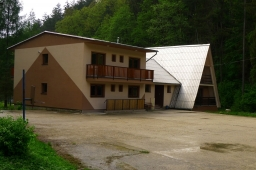# Accommodated 8189

One hundred sixty-nine pupils were accommodated in 45 rooms at the outdoor school. Some were triple, and some quadruple, and all were fully occupied. How many were triple and quadruple rooms there?

a =  11
b =  34

### Step-by-step explanation:

45 = a + b
169 = 3a + 4b

45 = a + b
169 = 3·a + 4·b

a+b = 45
3a+4b = 169

Pivot: Row 1 ↔ Row 2
3a+4b = 169
a+b = 45

Row 2 - 1/3 · Row 1 → Row 2
3a+4b = 169
-0.33b = -11.33

b = -11.33333333/-0.33333333 = 34
a = 169-4b/3 = 169-4 · 34/3 = 11

a = 11
b = 34

Our linear equations calculator calculates it.Did you find an error or inaccuracy? Feel free to write us. Thank you!

Tips for related online calculators
Do you have a linear equation or system of equations and looking for its solution? Or do you have a quadratic equation?